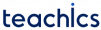Resistance transducer is one of the simplest and important groups of transducers. These are transducers whose electrical resistance is varied in accordance with the input quantity (measurand). Quantities like displacement, acceleration, force, pressure, temperature etc. can be transduced using this type of transducers.

## Basic Principle of Resistance Transducer

The basic principle of a resistance transducer is very simple.

Resistance of a conducting wire is given byWhere s is the resistivity of the material of the wire, l is the length of the wire and A is the area of cross-section.

Any measurand which varies any of these quantities like s,l or a, will result in a change in resistance of the wire. These change in resistance can be converted to a change in voltage by an electrical circuitry and thus a transducer is obtained.

The advantages of using a resistance transducer are,

• Quick response
• Low cost
• Suitable for both AC and DC
• Available in different sizes
• Easy operation

• Sliding contact can exhaust and may create noise in the output
• Huge power is necessary for many sliders.

Examples

• Potentiometer: The resistance is varied with the variation in their length and hence is used for measurement of displacement
• Strain gauge: Resistance is changed when applying strain and this property is used for measuring pressure, force etc.
• Thermistors (Resistance thermometers): Resistance changes with change in temperature and is used as thermometers.
Subscribe
Notify of